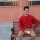Civil Engineering Questions
Question and answers for technical problems related to civil engineering subjects, jobs, courses and more
90 Members
Join this group to post and comment.Ali Hamza
Mechanical
24 Jan 2019

# What is Pressure??

PRESSURE

Fluid will exert a force normal to a solid boundary or any plane drawn through the fluid.

Since problems may involve bodies of fluids of indefinite extent and, in many cases, themagnitude of the force exerted on a small area of the boundary or plane may vary from placeto place, it is convenient to work in terms of the pressure p of the fluid, defined as the forceexerted per unit area. If the force exerted on each unit area of a boundary is the same, thepressure is said to be uniform.

If, as is more commonly the case, the pressure changes from point to point, we consider theelement of force δF normal to a small area δA surrounding the point under consideration.

Mean pressure, In the limit, as δA→0 (but remains large enough to preserve the concept of  the fluid as a continuum), Pressure at a point,

Units:

newtons per square metre (N m−2 ) (Note that an alternative metric unit is the bar; 1 bar = 105 N m−2)

Dimensions: ML−1T −21mo ago

P= F/A

Force

Area

Pressure is inversely proportional to Volume.

These two concepts helps me alot while I was deal with FM and other subjects in core engineering as well as applied engineering classes.